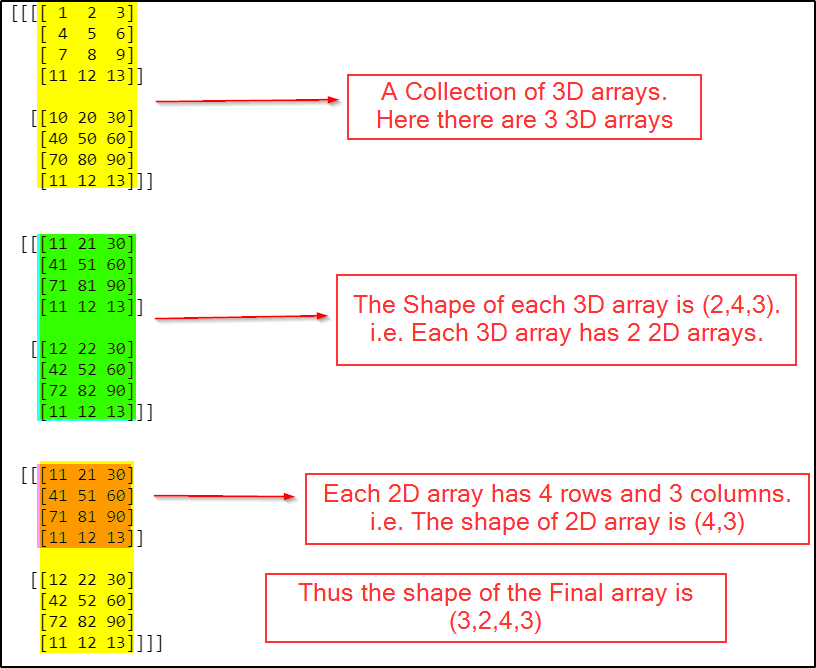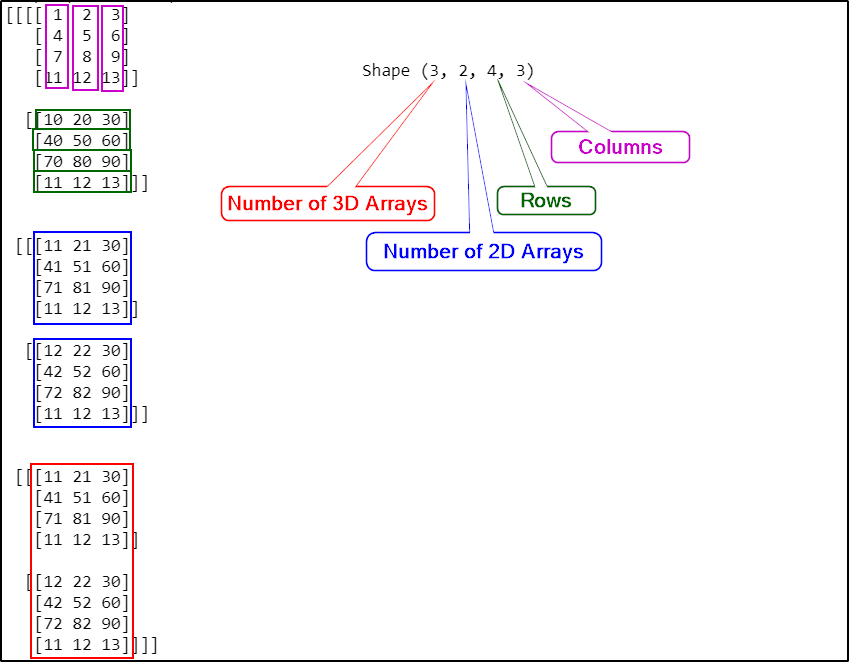# Python — Numpy — Understanding Arrays & DimensionsSource: Photo by Faris Mohammed on Unsplash

Numpy is a python library used for computing scientific/mathematical data.

### Numpy usages are in:

• Numerical Analysis
• Linear algebra
• Matrix computations
• Including the above concepts, Numpy provides an important feature called Arrays
• Even though it is similar to python List, Array has advantages over List.

### Advantages of Arrays are:

• Memory Efficient
• Faster
• Convenient

Below is the code to create a new Numpy Array.

The array() method takes a list of items that go into the array.

The above array is a one-dimensional array of 4 elements.

The concept of rows and columns applies to Numpy Arrays only if the dimension is more than 1.

For a 1 dimensional array is more like a list, there is no concept of rows and columns, so the shape just displays the number of items in the list.

Output:

In the above output, you can see that the shape is 3 rows and 4 columns.

Also, you can notice that the array started with 2 square brackets [[ and ended with 2 square brackets ]].

This will make us understand easily that it is a 2D array.

A 2D array is just a collection of 1D arrays.

Similarly, a 3D array is a collection of 2D arrays and goes on.

Let’s see a 3D array now.

Output:

### In the above result, the shape is defined as:

• Here there are 2 3D arrays which become the First number,
• The second number is Rows
• The third number is columns

Let’s see another example for more understanding.

Output:

Let us see an example of 4D array now.

Output:Image created by Author

To understand more about each number in the shape, please see the picture below.Image created by Author

Shape of the above array is: 4D which is a collection of three 3D arrays

• The first Number is the number of 3d Arrays,
• The second number is the number of 2d Arrays,
• The third Number is Rows,
• The fourth Number is columns.

P.S: I am trying my best to give you the Jupyter Notebook cells as good as possible. Hopefully, It will be good in my next articles.

### Conclusion:

In this article, we have gone through the various dimensions of the array and its shape.

In my upcoming article, we will be reviewing how easy it is to use Numpy arrays when it comes to matrix manipulations using Numpy Arrays and changing the shape of an array.

0

## 2 Replies to “Python — Numpy — Understanding Arrays & Dimensions”

1.Anuradha Perumalsamy says:

Well explained.

0
1.Asha Ponraj says:

Thank you 😊

0